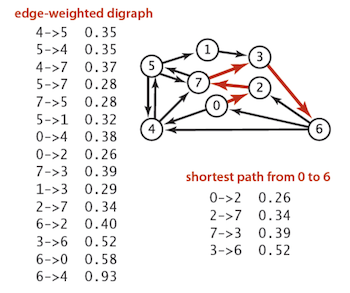## Dijkstra's Shortest Path Algorithm

Given an edge-weighted digraph, we often wish to find the shortest (directed) path from a given vertex $v$ to another given vertex $w$. As an example, the shortest path from vertex $0$ to vertex $6$ in the given digraph is shown below:The above task is called the single-pair shortest path problem. There are a few variations on this problem, however, that will interest us:

• The single-source shortest path problem, in which one needs to find shortest paths from a source vertex $v$ to all other vertices in the graph. Interestingly, there are no known algorithms for the single-pair problem that run asymptotically faster than the best single-source algorithm in the worst case.

• The single-destination shortest path problem, in which we have to find shortest paths from all vertices in the directed graph to a single destination vertex v. Note, this can be reduced to the single-source shortest path problem by reversing the edges in the directed graph.

• The all-pairs shortest path problem, in which we have to find shortest paths between every pair of vertices $v$ and $w$ in the graph.

Dijkstra's developed an algorithm, published in 1959, which grows paths from a given source vertex using a "greedy approach" and finds all shortest paths to this source vertex -- thereby solving the aforementioned single-source problem, the single-pair problem, and (by reduction) the single-destination problem as well. The essential components of this algorithm are given below.

Dijkstra's Shortest Path Algorithm
1. Assign to every vertex a value which will store an associated distance. For the source vertex, make this distance zero. For every other vertex, initially make this distance infinite.

2. Mark all nodes as unvisited.

3. Set the "current vertex" initially to be the source vertex.

4. Repeat the following until all vertices have been visited:

1. For the current vertex, consider all its unvisited neighbors and calculate their tentative distance (i.e., the sum of the distance associated with the current vertex plus the distance between the current vertex and the unvisited neighbor in question).

For each adjacent neighbor to the current vertex, if this distance is less than the previously recorded distance that vertex, overwrite the previously recorded distance with this smaller distance (edge relaxation) and then mark the current vertex as visited.

2. Set the unvisited node with the smallest distance from the source node as the next "current node"

Note, the term "edge relaxation" as used above comes from an analogy between the estimate of the length of the shortest path and the length of a helical tension spring, which is not designed for compression. Initially, the cost of the shortest path is an overestimate, likened to a stretched out spring. As shorter paths are found, the estimated cost is lowered, and the spring is relaxed. Eventually, the shortest path, if one exists, is found and the spring has been relaxed to its resting length.)